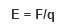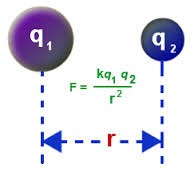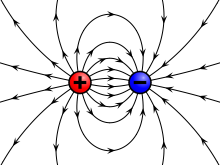Click to Chat

1800-1023-196

+91-120-4616500

CART 0

• 0

MY CART (5)

Use Coupon: CART20 and get 20% off on all online Study Material

ITEM
DETAILS
MRP
DISCOUNT
FINAL PRICE
Total Price: Rs.

There are no items in this cart.
Continue Shopping• Complete JEE Main/Advanced Course and Test Series
• OFFERED PRICE: Rs. 15,900
• View Details

```Introduction to Electric Charge and Fields

Table of Content

What is Electric Charge?

Example of Electric Charge

Properties of Electric Charge

What is Electric Field?

How to calculate SI Unit of any Physical Quantity?

For Example

How to calculate the Direction of E?

Diagram of E in terms of two Charges

What is Electric Charge?Electric Charge is that physical property of matter due to which the other matter experience a force when matters are placed in electromagnetic field. Electric charge is also known as Charge, Electrical Charge and Electrostatic Charge. It is denoted by symbol ‘q’. It is a scalar quantity as charge has only magnitude and no direction.

The two types of charges exist in nature: Positive and Negative Charge.

Same charge repels each other and opposite charge attract each other. In the figure given below, we can see that like charge are repelling each other, while opposites are attracting each other.

Example of Electric Charge

We know that in a nucleus proton and neutron exists while electron revolve around the nucleus. Proton (p+) have positive charge and electron (e-) have negative charge. As symbol of proton is (p+) so proton carrying positive charge and symbol of electron is (e-) so electron carrying negative charge. As number of proton and electron in a nucleus are equal so net charge on nucleus is zero.

For existing the net charge on a body the sum of positive and negative charge should be equal to zero.

Properties of Electric Charge

Charge is measured in terms of Coulombs (C)

Charge is a scalar quantity

Charge can be positive or negative

Charge of proton and electron have same in magnitude but opposite in sign. Proton have charge 1.6 * 10-19 C while electron have -1.6 * 10-19 C

Charge is conserved while transferring.

Charge is quantized in nature.

Example: Q = n e  where e, is the electric unit of charge and its value is 1.6 * 10-19. C and n is a integer it may be positive or negative.

What is Electric Field?

It is the region around a charge particle or object due to which another charge particle or object experience force. Electric Field is a vector quantity so we must consider the sign in numerical.

Formula of Electric FieldUnit of electric field: E = N/CAs, unit of force is Newton (N)  and charge is Coulomb ( C),  So unit of electric field is N/C.

Note: While writing the unit 1st letter should be capital.

In the above formula F is the force and q is the charge. F is calculated in terms of coulombs law.

Coulombs law is stated as force acting on a charge particle due to another charge particle is directly proportional to product of their charges and inversely proportional to square of distance between the charges.

From the above definition, of coulomb’s forceWhere, k is the proportionality constant and its value is 9 * 109 Nm2/C2. It is different for different medium.

SI unit of F is = N m2 C2/C2 m2

So, on solving above equation we get F = Newton (N)

How to calculate SI Unit of any Physical Quantity?

Quality

SI unit

Charge

Coulomb (C)

Mass

Kilogram (Kg)

Distance

Meter

Force

Newton (N)

These four quantities are the basic quantities with the help of these quantities we can find the SI unit of any other physical quantity.

For Example

SI unit of Electric Field

We, know that formula of E is = F/q

So, SI unit of F is Newton and Charge is Coulomb.

E = N/C

How to calculate the Direction of E?

Direction of Electric Field is always from positive charge to negative charge. In terms of single positive charge Electric field move away from the positive charge as shown in the first part of the diagram and in terms of negative charge Electric field move towards the negative charge as shown in the second part of the diagram.In the above diagram, we can see that in terms of positive charge E is moving away from positive charge Similarly in terms of negative charge E moving away from negative charge.

Diagram of E in terms of two ChargesIn this diagram, all the three directions are used. Firstly, Electric field goes from Positive charge to negative charge. Secondly, In terms of positive charge field lines are moving away from positive charge and in terms of negative charge field lines are coming towards the charge.

Q1. Two insulated charge sphere A and B of identical size having charges qA and -3qA respectively. When they are brought in contact with each other and then separated what are the new charges on them?

Sol. As two charges are of opposite sign, they will attract each other so there will be the simple addition of charge.

New charge on them is  Q = (qA) + (-3qA / 2)

Q = -qA

Q2.  What is the basic condition for existing the net charge on a body?

Sol. The basic condition for existing the net charge on a body is that number of positive and negative charge should not be equal to zero.

Q3. What is the Charge on Electron?

Sol.  Charge on electron is -1.6 *10-19 C.

Q4.  Is the force acting between two point charges q1 and q2 kept at some distance apart, attracting or repulsive (a) q1 q2 > 0 (b) ) q1 q2 < 0 ?

Sol.

(a) If q1 q2 > 0 then the force is repulsive because if both the charges are positive and negative (same charges) only then their product is greater than zero. We know that same charges always repel each other.

(b) If q1 q2 < 0 then the force is attractive because one charge will be positive and other will be negative and opposite charge attract each other.

Q5. What is the SI unit of Electric Field?

Sol. SI unit of electric field is N/C.

Q6. What is the Dimensional Formula of Electric Field?

Sol. The dimensional formula of electric field is [MLT-2C-1].

Q7. If two charges having equal magnitude are separated by unit distance and exert a force of 15 N on each other. What will be the force exerted by the same charges kept at same distance but the charges are placed in water?

Sol.

F1 = 15 N, r1 = 1 meter, k air = 1 , q1 = q2 = q

F2 = 15 N, r2 = 1 meter, k water = 80

F1 = k air q1 q2 / r2,      F2 = k water q1 q2 / r2

F2 = k water q1 q2 / r2

F2 = 80 * q2/ 1

F2 = 80 q2 N.

Q8. Two spheres of radius r1 and r2 are connected by a wire. What will be the ratio of their electric field?

Sol.  We know that F= k q1 q2/r2 and E = F/q.

So, E1/E2 = r22/r12.

Watch this Video for more reference

```### Course Features

• 728 Video Lectures
• Revision Notes
• Previous Year Papers
• Mind Map
• Study Planner
• NCERT Solutions
• Discussion Forum
• Test paper with Video Solution